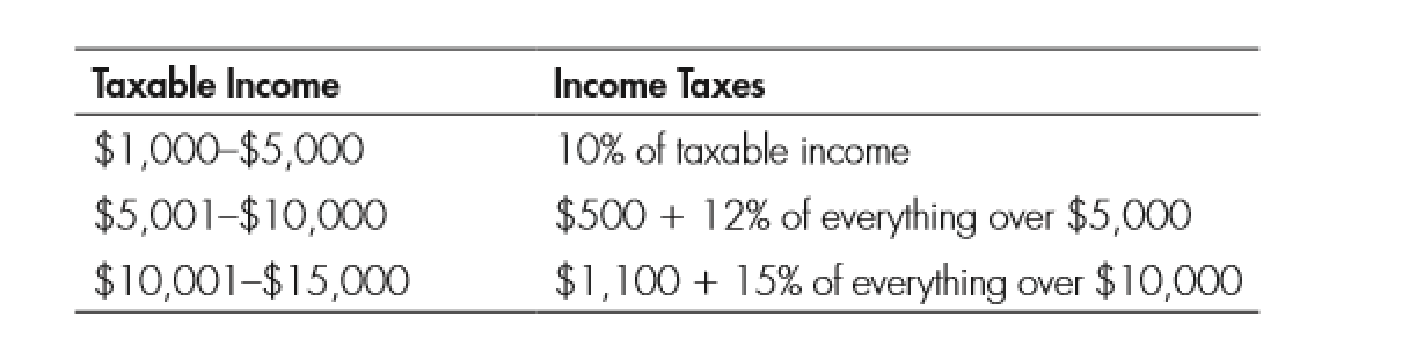Chapter 11, Problem 1WNG

Chapter
Section
Textbook Problem

Use the following table to answer questions 1–4:1. If a person’s income is $6,000, how much does he pay in taxes? To determine Identify the taxable amount. Explanation As per the given table, people lie under the income range between$5,001 and $10,000 and have to pay$500 plus 12% of everything over $5,000 as tax. Since the income is$6,000, 12% of $1,000 ($6,000-$5,000) is$120

Still sussing out bartleby?

Check out a sample textbook solution.

See a sample solution

The Solution to Your Study Problems

Bartleby provides explanations to thousands of textbook problems written by our experts, many with advanced degrees!

Get Started

Find more solutions based on key concepts